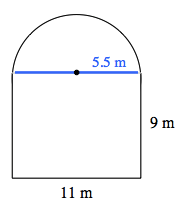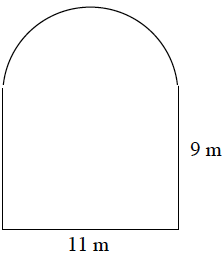### Home > MC1 > Chapter 10 > Lesson 10.2.3 > Problem10-107

10-107.

The shape below is composed of a rectangle and a semi-circle (half of a circle).

1. Find the perimeter.The perimeter of a semi-circle is half of the circumference of a circle.

If the semi-circle were made into a complete circle, its circumference would be 11π.

46.27 meters

2. Find the area.

Find the area of the semi-circle and the area of the rectangle separately, then add them together to find the total area.